### Brazuca, a Pogorelov's ball

posted by @ulaulaman about #Brazuca #geometry #WorldCup2014 #Brazil2014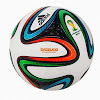Brazuca is the ball of the World Cup 2014. The particular pattern of its surface is a consequence of the Pogorelov's theorem about convex polyhedron:
A domain is convex if the segment joining any two of its points is completely contained within the field.
Now consider two convex domains in the plane whose boundaries are the same length.(1)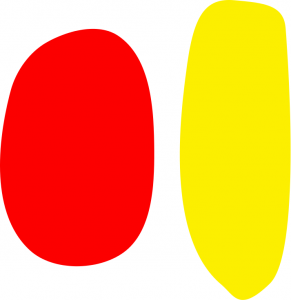Now we can create a solid using the two previous domains: we must simlply connect every point of one boundary with a point of the other boundary, obtaining a convex polyhedron, like showed by Pogorelov in 1970s.
The object you have built consists of two developable surfaces glued together on edge.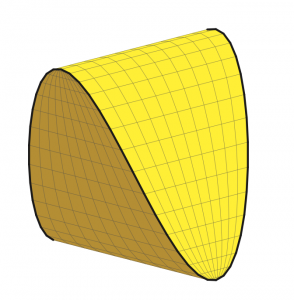Instead of using two domains, you can, for example, start from six convex domains as the "square faces" of a cube. On the edges of each of these areas, you choose four points, as the vertices of the "square". We assume that the four "corners" that you have chosen are like the vertices, that is to say that the domains have angles in these points.(1)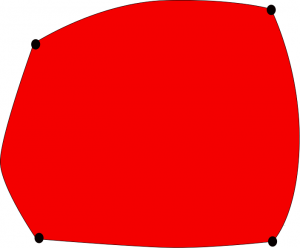Let us to assume that the legths of all "curved edges" are the same(1): you can paste any domains like in a cube.
It's still necessary another assumption: each vertex of a cube is common to three sides. The sum of the corresponding angles must be less than or equal to 360 degrees.
Pogorelov's theorem guarantees that, under these assumptions, it works.
You make a kind of cube, whose edges are curved, and whose six faces are developable and not necessarily planar.
It is not needed to apply the theorem that the domains are convex. The important condition is that when you glue together two points, the sum of the curvatures of the two fields at each point of contact is positive. In less precise terms, we must pick a concavity with a higher convexity.(1)
In this way it is possible to abtain the Brazuca: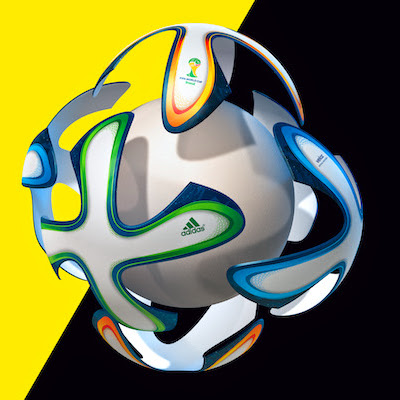It's interesting to observe that a very similar results was reached by William Thurston and Kelly Delp(2)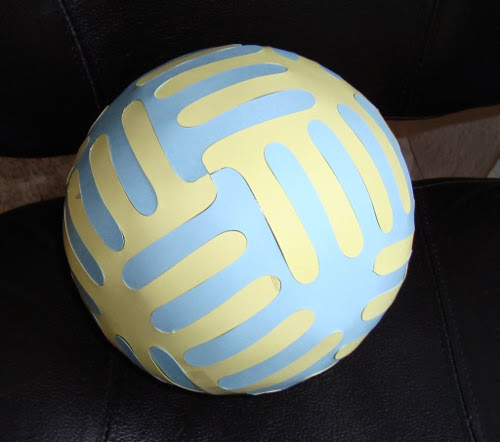(1) Étienne Ghys. Le Brazuca, le ballon cubique de la Coupe du monde
(2) Kelly Delp, William Thurston. Playing with Surfaces: Spheres, Monkey Pants and Zippergons. Bridges Coimbra Conference Proceedings (2011), 1-8